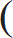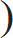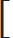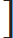# Surds and Indices - Aptitude test, questions, shortcuts, solved example videos

Video on Surds and Indices - shortcuts, tips and tricks

## Surds and Indices

Before solving the numerical on this chapter make sure that you are perfect with the chapter of square and square roots, simplification, linear and quadratic equations and decimal fractions.

Important Terms

Surd: Number which cannot be expressed in the fraction form of two integers is called as surd. Hence, the numbers in the form of 3, 32, ……. nx
 For example: 1 can be written as 1 but 3 cannot be written in the form of fraction 9 3
Irrational numbers which contain the radical sign (     ) are called as surds.

Indices: Indices refers to the power to which a number is raised. Index is used to show that a number is repeatedly multiplied by itself.

For example: a3 is a number with an index of 3 and base ‘a’. It is called as “a to the power of 3”

Quick Tips and Tricks

1) The laws of indices and surds are to be remembered to solve problems on surds and indices.

Laws of Indices

1) xm × xn = am+n

2) (xm)n = xmn

3) (xy) n = xnyn
 4) xm = xm – n xn
 5)xn = xn y yn
 6) x–1 = 1 x

Laws of Surds

1) nx = x(1/n)

2) nxy = nx × ny
 n(x/y) = nx ny

4) (nx)n = X

5) mnx = mnx

6) (nx)m = (nxm)

2) Expressing a number in radical form

Example: l x(m/n) l = nxm

The exponential form l x(m/n) l is expressed in radical form as nxm

Important points to Remember

1) Any number raised to the power zero is always equals to one. (Eg: x 0 = 1)
2) Surd nx can be simplified if factor of x is a perfect square
3) If denominator in a fraction has any surds, then rationalize the denominator by multiplying both numerator and denominator by a conjugate surd.
4) Every surd is an irrational number, but every irrational number is not a surd.
5) The conjugate of (2 + 7i) is (2 – 7i)
6) Different expressions can be simplified by rationalizing the denominator and eliminating the surd.

Rationalizing the denominator:

To rationalize the denominator 7 multiply with its conjugate to both numerator and denominator
 Example 1: 1 = 1 × 7 = 7 7 7 7 7

 Example 2: 1 = 1 × 7 – 3 = 7 – 3 7 + 3 7 + 3 7 – 3 4

Types of Questions:

Type 1: Simplify the given expressions using laws of indices and surds.

Q 1. (1331)– (2/3)
 a. – 1 11

 b. – 11 121

 c. 1 121

 d. 121 11
View solution

Correct Option: (c)

Cube root of 1331 is 11. Therefore,
(113)– (2/3)

Hint:
Remember the law of indices (xm)n = xmn

(11) – 3 × (2/3) = 11–2

Hint:

 x–1 = 1 x

 Hence, 11–2 = 1 = 1 112 121

 Q 2. (32)(n/5) × 22n + 1 4n × 2n – 1

a. 4
b. 8
c. 2n
d. 2n + 1
View solution

Correct Option: (a)

32 = 25 and 4 = 22
Substituting these values, we get

 (32)(n/5) × 22n + 1 = (25)(n/5) × 22n + 1 4n × 2n – 1 (22)n × 2n – 1

Hint:
Laws of indices (xm)n = xmn and xm × xn = am + n
 (25)(n/5) × 22n + 1 = (2) 5 × (n/5) × 22n + 1 = 2n × 22n + 1 (22)n × 2n – 1 22n × 2n – 1 22n × 2a – 1

In the expression, bases are same, hence add the indices.
 2n × 22n + 1 = 2n + 2n + 1 = 23n + 1 22n × 2n – 1 22n + n – 1 23n – 1

 xm = xm – n xn

 23n + 1 = 23n + 1 – (3n – 1) = 22 = 4 23n – 1

 Q 3. Find the value of 1 + 1 + 1 125–(2/3) 625–(3/4) 729–(3/6)

a. 132
b. 177
c. 185
d. 225
View solution

Correct Option: (b)

53 = 125, 54 = 625, 36 = 729

 1 + 1 + 1 (53)–(2/3) (54)–(3/4) (36)–(3/6)

Hint:
Law of indices (xm)n = xmn
 1 + 1 + 1 (5)–2 (5)–3 (3)–3

Therefore,
 1 + 1 + 1 = 52 + 53 + 33 = 177 (5)–2 (5)–3 (3)–3

Type 2: Find the value of x in the given expression.

 Q 4. If x = 5 + 26, then find the value ofx – 1x

a. 26
b. 6
c. 8
d. 6
View solution

Correct Option: (c)

 We have to find the value ofx – 1firstly simplify this term without any radical sign. x
Hence, square the given expressionx – 12 = x – 2 × x × 1 + 1 = x – 2 + 1 - - - -[(a – b)2 = a2 – 2ab + b2] x x x x

Now, substitute the value of x = 5 + 26 in the simplified expression x – 2 + (1/x)
 x + 1 – 2 = 5 + 26 + 1 – 2 x 5 + 26

To rationalize the denominator, multiply numerator and denominator by its conjugate 5 – 26
 = 5 + 26 + 1 × 5 – 26 – 2 5 + 26 5 – 26

 = 5 + 26 + 5 – 26 – 2 - - - (a + b)(a – b) = (a2 – b2) 25 – 24

= 5 + 5 – 2
= 8

Q 5. If 2x × 8(1/4) = 2(1/4) then find the value of x
 a. – 1 2
 b. 1 2
 c. 1 4
 d. – 1 4
View solution

Correct Option: (a)

2x × 8(1/4) = 2(1/4)

As bases are not equal we cannot add the indices, hence first convert all the numbers with same base.
2x × (23)(1/8) = 2(1/4)

Hint:
Law of Indices (xm)n = xmn

2x × 2(3/4) = 2(1/4)

2[x + (3/4)] = 2(1/4)

 x + 3 = 1 4 4

 x = 1 – 3 = –2 = –1 4 4 4 2

Q 6. If 9x – 9x – 1 = 648, then find the value of xx

a. 4
b. 9
c. 27
d. 64
View solution

Correct Option: (d)

Hint:
xm × xn = xm + n

9x – 9x – 1 = 648

9x – 1 (9 – 1) = 648

9x – 1 = (648/8) = 81

9x – 1 = 92

x – 1 = 2

x = 2 + 1 = 3

xx = 33 = 27

Alternate solution:

Select the given options and substitute the value of x = 5, 6, 1.5 and 3 in the given expression.

Substituting value of x = 3,
9x – 9x – 1 = ?
93 – 93 – 1 = 648
Value of x = 3

xx = 33 = 27

Q 7. If 4 (x – y) = 64 and 4 (x + y) = 1024, then find the value of x.

a. 3
b. 1
c. 6
d. 4
View solution

Correct Option: (d)

4 (x – y) = 64
4 (x – y) = 64 = 43
Equation 1) x – y = 3

4 (x + y) = 1024 = 45
Equation 2) x + y = 5

Solving equation (1) and (2), we get

x = 4 and y = 1

Crosscheck the answers by substituting the values of x and y in the given expression.

4 (4 – 1) = 43 = 64 and 4 (4 + 1) = 45 = 1024

Hence, the answers x = 4 and y = 1 are correct.

Q 8. If a and b are whole numbers such that ab = 121, then find the value of (a – 1)b + 1

a. 0
b. 10
c. 102
d. 103
View solution

Correct Option: (d)

121 = 112 , hence value of a = 11 and b = 2 can be considered.

Therefore, the value of (a – 1)b + 1 = (11 – 1) 2 + 1= 103

Practice questions on Surds and Indices
Ratio and Proportion - Aptitude test, questions, shortcuts, solved example videos
Ratio and Proportion - Quantitative aptitude tutorial with easy tricks, tips, short cuts explaining the concepts. Online aptitude preparation material with practice question bank, examples, solutions and explanations. Video lectures to prepare quantitative aptitude for placement tests, competitive exams like MBA, Bank exams, RBI, IBPS, SSC, SBI, RRB, Railway, LIC, MAT. Very useful for freshers, engineers, software developers taking entrance exams. Learn and take practice tests!
Problems on Chain Rule - Aptitude test, questions, shortcuts, solved example videos
Problems on Chain Rule - Quantitative aptitude tutorial with easy tricks, tips, short cuts explaining the concepts. Online aptitude preparation material with practice question bank, examples, solutions and explanations. Video lectures to prepare quantitative aptitude for placement tests, competitive exams like MBA, Bank exams, RBI, IBPS, SSC, SBI, RRB, Railway, LIC, MAT. Very useful for freshers, engineers, software developers taking entrance exams. Learn and take practice tests!
Pipes and Cisterns - Aptitude test, questions, shortcuts, solved example videos
Pipes & Cisterns - Quantitative aptitude tutorial with easy tricks, tips, short cuts explaining the concepts. Online aptitude preparation material with practice question bank, examples, solutions and explanations. Video lectures to prepare quantitative aptitude for placement tests and competitive exams like MBA, Bank exams, RBI, IBPS, SSC, SBI, RRB, Railway, LIC, MAT. Very useful for freshers, engineers, software developers taking entrance exams. Learn and take practice tests!